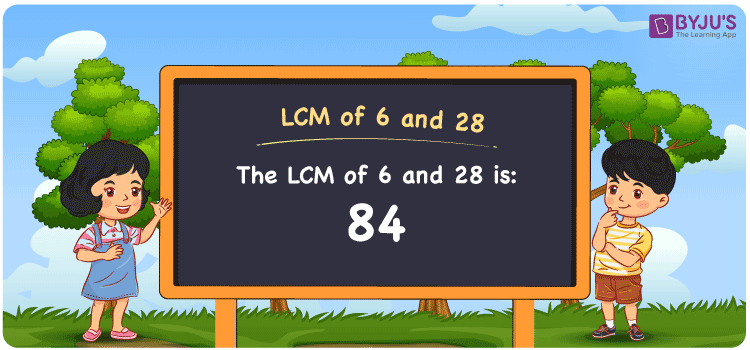# LCM of 6 and 28

LCM of 6 and 28 is 84. From the multiples of 6 and 28, the number which is evenly divisible by the two numbers gives the LCM value. Least common multiple of 6 and 28 is the common multiple which is obtained using the multiplication operation. (6, 12, 18, 24, 30, ….) and (28, 56, 84, 112, 140, ….) are the multiples of 6 and 28. The LCM of two numbers is found using methods like listing multiples, prime factorization and division.

Also read: Least common multiple

## What is LCM of 6 and 28?

The answer to this question is 84. The LCM of 6 and 28 using various methods is shown in this article for your reference. The LCM of two non-zero integers, 6 and 28, is the smallest positive integer 84 which is divisible by both 6 and 28 with no remainder.## How to Find LCM of 6 and 28?

LCM of 6 and 28 can be found using three methods:

• Prime Factorisation
• Division method
• Listing the multiples

### LCM of 6 and 28 Using Prime Factorisation Method

The prime factorisation of 6 and 28, respectively, is given by:

6 = 2¹ x 3¹

28 = 2 x 2 x 7 = 2² x 7¹

LCM (6, 28) = 84

### LCM of 6 and 28 Using Division Method

We’ll divide the numbers (6, 28) by their prime factors to get the LCM of 6 and 28 using the division method (preferably common). The LCM of 6 and 28 is calculated by multiplying these divisors.

 2 6 28 2 3 14 3 3 7 7 1 7 x 1 1

No further division can be done.

Hence, LCM (6, 28) = 84

### LCM of 6 and 28 Using Listing the Multiples

To calculate the LCM of 6 and 28 by listing out the common multiples, list the multiples as shown below

Multiples of 6 = 6, 12, 18, 24, 30, 36, 42, 48, 54, 60, 66, 72, 78, 84, ….

Multiples of 28 = 28, 56, 84, 112, 140, 168, 196, …..

LCM (6, 28) = 84

## Video Lesson on Applications of LCM## LCM of 6 and 28 Solved Examples

Question: The LCM and GCD of 6 and 28 is 84 and 2. Find the other number if one number is 6.

Solution:

Consider n as the other number

LCM x GCD = 6 x n

n = (LCM x GCD)/6

n = (28 x 6)/ 6

n = 28

Hence, the other number is 28.

## Frequently Asked Questions on LCM of 6 and 28

### List the methods used to determine the LCM of 6 and 28.

The methods used to determine the LCM of 6 and 28 are Prime Factorisation, Division method and Listing the multiples.

### Using the prime factorisation method, what is the LCM of 6 and 28?

We should analyze the factors first to determine the LCM

6 = 2¹ x 3¹

28 = 2 x 2 x 7 = 2² x 7¹

LCM is the product of prime factors raised to the highest exponent among 6 and 28.

LCM of 6 and 28 = 84

### Calculate the GCF if the LCM of 6 and 28 is 84.

LCM x GCF = 6 x 28

As the LCM = 84

84 x GCF = 168

GCF = 168/84 = 2

### What is the LCM of 6 and 28?

The LCM of 6 and 28 is 84.

### In 15, 25, 45, 84, find the LCM of 6 and 28.

The LCM of 6 and 28 is the value which is evenly divisible by the given numbers. 84 is the number which satisfies this condition.